# pls do all Page 6 of 14 22) Identify a good buffer. ) small amounts of both a weak acid and its conjugate base B) significant amounts of both a strong acid and a strong bso C) small amounts of bot...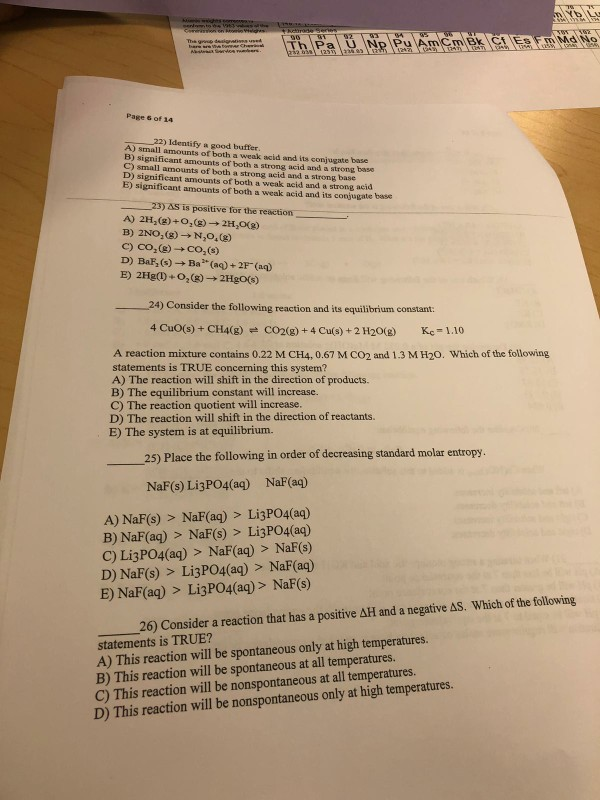pls do all

Page 6 of 14 22) Identify a good buffer. ) small amounts of both a weak acid and its conjugate base B) significant amounts of both a strong acid and a strong bso C) small amounts of both a strong acid and a strong base D) significant amounts of both a weak acid and a strong acid E) significant amounts of both a weak acid and its conjugate base 23) AS is positive for the reaction A) 2H2(g) +02(g)→2H2O(g) C) CO2(g) . D) BaF,(s)- Co,(s) Ba(a)+2F(a) 24) Consider the following reaction and its equilibrium constant: 4 CuO(s) + CH4(g)-CO2(g) + 4 Cu(s) +2 H2O(g) Kc = 1.10 A reaction mixture contains 0.22 M CH4, 0.67 M CO2 and 1.3 M H20. Which of the following statements is TRUE concerning this system? A) The reaction will shift in the direction of products. B) The equilibrium constant will increase. C) The reaction quotient will increase. D) The reaction will shift in the direction of reactants. E) The system is at equilibrium. 25) Place the following in order of decreasing standard molar entropy NaF(s) LigPO4(aq) NaF(aq) A) NaF(s) > NaF(aq) > Li3PO4(aq) B) NaF(aq) > NaF(s) > Li3PO4(aq) C) Li3PO4(aq) > NaF(aq) > NaF(s) D) NaF(s) > Li3PO4(aq) > NaF(aq) E) NaF(aq) > Li3PO4(aq)> NaF(s) 26) Consider a reaction that has a positive AH and a negative AS. Which of the following statements is TRUE? A) This reaction will be spontaneous only at high temperatures. B) This reaction will be spontaneous at all temperatures. C) This reaction will be nonspontaneous at all temperatures. D) This reaction will be nonspontaneous only at high temperatures.

22) Answer is E). Strong acid and base don't act as good buffer.

23) Answer is D). Because product is aqueous which has high entropy than solid reactant.

Reaction quotient,Q = [CO2][H2O]2/[CH4] = 0.67×(1.3)2/0.22

Q = 5.147

Since Q>K hence reaction go backward

25) Answer is C). Solid has low entropy and higher the ions higher entropy.

26) Answer is C). As ∆G = ∆H-T∆S so, ∆G is positive hence reaction is non-spontaneous at all temperature.

##### Add Answer of: pls do all Page 6 of 14 22) Identify a good buffer. ) small amounts of both a weak acid and its conjugate base B) significant amounts of both a strong acid and a strong bso C) small amounts of bot...
Similar Homework Help Questions
• ### A buffer solution is able to maintain a constant pH when small amounts of acid or...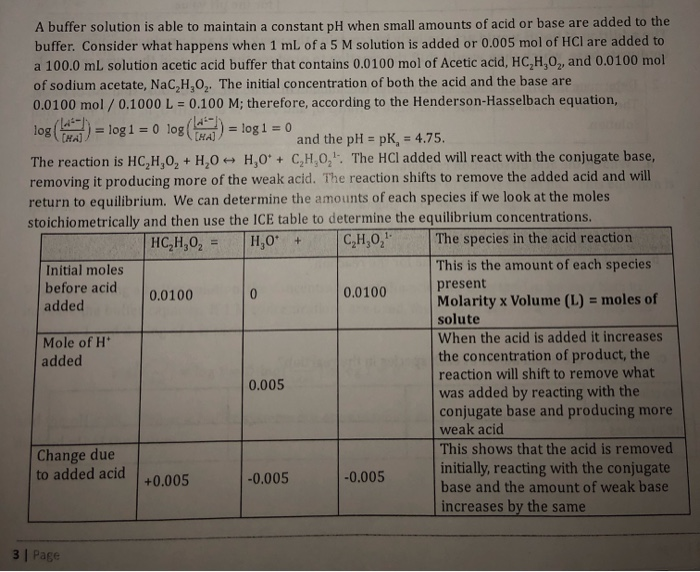A buffer solution is able to maintain a constant pH when small amounts of acid or base are added to the buffer. Consider what happens when 1 mL of a 5 M solution is added or 0.005 mol of HCl are added to a 100.0 mL solution acetic acid buffer that contains 0.0100 mol of Acetic acid, HC,H,O,, and 0.0100 mol of sodium acetate, NaC,H,O,. The initial concentration of both the acid and the base are 0.0100 mol/ 0.1000 L...

• ### CHE 172 Acid-Base Equilibrium Worksheet 1. Identify the conjugate pairs in the following reaction: HC2H302(aq) +...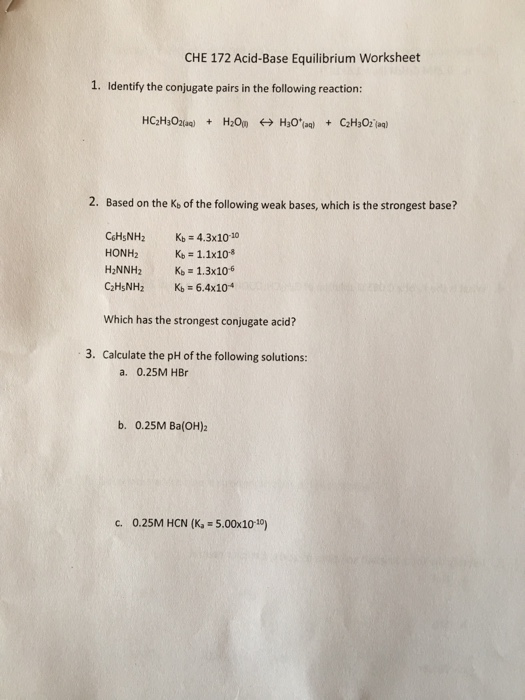CHE 172 Acid-Base Equilibrium Worksheet 1. Identify the conjugate pairs in the following reaction: HC2H302(aq) + H2O + H20*24) + CH3O2 (aq) 2. Based on the Kb of the following weak bases, which is the strongest base? C6H5NH2 HONH2 H2NNH2 C2H5NH2 Kb = 4.3x10-10 Ko = 1.1x10-8 Kb = 1.3x106 Kb = 6.4x104 Which has the strongest conjugate acid? 3. Calculate the pH of the following solutions: a. 0.25M HBO b. 0.25M Ba(OH)2 C. 0.25M HCN (K. = 5.00x10-10) d....

• ### Question two A buffer solution is able to maintain a constant pH when small amounts of...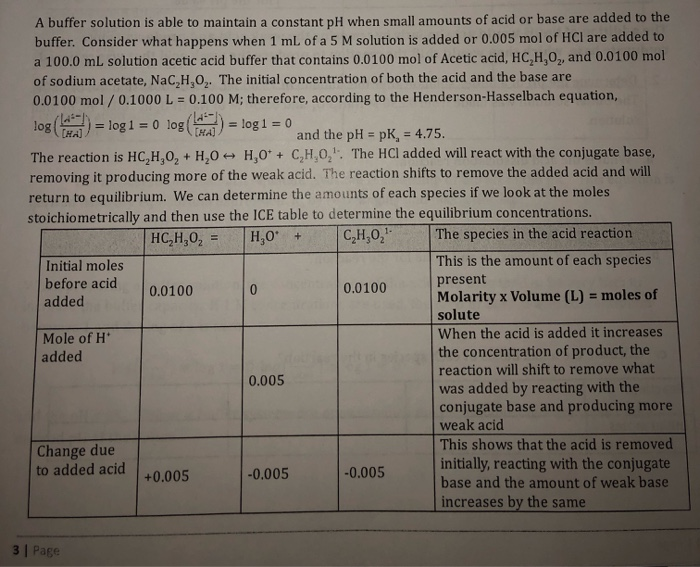Question two A buffer solution is able to maintain a constant pH when small amounts of acid or base are added to the buffer. Consider what happens when 1 mL of a 5 M solution is added or 0.005 mol of HCl are added to a 100.0 ml solution acetic acid buffer that contains 0.0100 mol of Acetic acid, HC,H,O,, and 0.0100 mol of sodium acetate, NaC,H,O2. The initial concentration of both the acid and the base are 0.0100 mol/0.1000...

• ### CH,OH)02 [CO2]2(H20] c. Qc 19. A is a proton donor. a. Bronsted Base b. Bronsted Acid...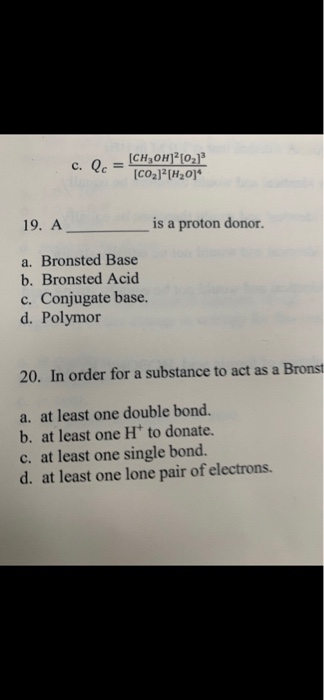CH,OH)02 [CO2]2(H20] c. Qc 19. A is a proton donor. a. Bronsted Base b. Bronsted Acid c. Conjugate base d. Polymor 20. In order for a substance to act as a Bronst a. at least one double bond. b. at least one H* to donate. C. at least one single bond. d. at least one lone pair of electrons. 16. The Gibhbs energy for the reaction beiow is CH ( 50(p-3C0p-4no a. 0 b. -2108 kJmol c. -608.1 kmol d....

• ### i want answer to all questions please Answer: 29) Consider the following generalized buffer solution equilibrium: When a small amount of a strong base such as sodium hydroxide is added to the so...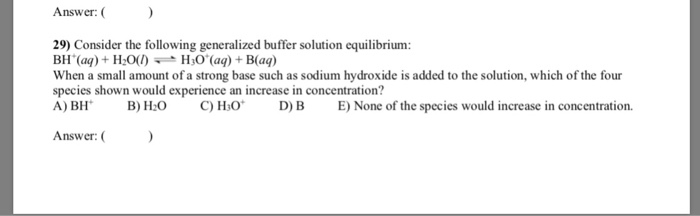i want answer to all questions please Answer: 29) Consider the following generalized buffer solution equilibrium: When a small amount of a strong base such as sodium hydroxide is added to the solution, which of the four species shown would experience an increase in concentration? A) BH B)H C) HO D)B E None of the species would increase in concentration. Answer: ( 30) What is true about a solution whose pH is less than 7 at 25°C? A) It has...

• ### 36) Which one of the following is not a strong acid? (or a weak electrolyte?) a) HNO )HCI HI )HF e) HCIO Answer: 37) The is the conjugate species that remains after an acid donates a proton is ca...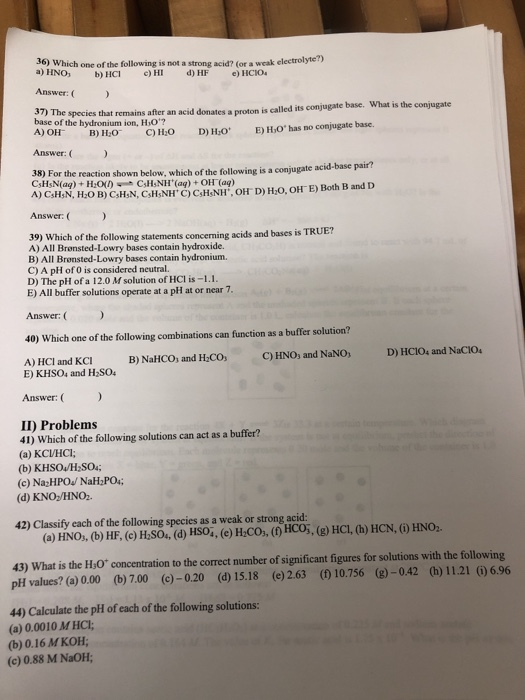36) Which one of the following is not a strong acid? (or a weak electrolyte?) a) HNO )HCI HI )HF e) HCIO Answer: 37) The is the conjugate species that remains after an acid donates a proton is called its conjugate base. What base of the hydronium ion, HO? B)HO C)HO D)H E HO'has no conjugate base. Answer: acid-base pair? 38) For the reaction shown below, which of the following is a conjugate acid A)CHsN, H:0 B) CsH,N, CsH,NH' C)...

• ### 1 . If a buffer solution is 0.260 M in a weak acid (?a=8.3×10−5)and 0.480 M in its conjugate base, what is the pH? pH= 2...

1 . If a buffer solution is 0.260 M in a weak acid (?a=8.3×10−5)and 0.480 M in its conjugate base, what is the pH? pH= 2. If a buffer solution is 0.200 M in a weak base (?b=5.0×10−5) and 0.530 M in its conjugate acid, what is the ph 3. Phosphoric acid is a triprotic acid (?a1=6.9×10−3, ?a2=6.2×10−8 , and ?a3=4.8×10−13 To find the pH of a buffer composed of H2PO4 - (aq) ) and HPO4 2− (aq) , which...

• ### pls do all Page 8 of 14 Short Answer (116 points) SHOw ALL wORKI!!!!I if you do not show your partial eredit!!!! work, there will be no 31. (15 points) Predict/Circle your choice for how each of t...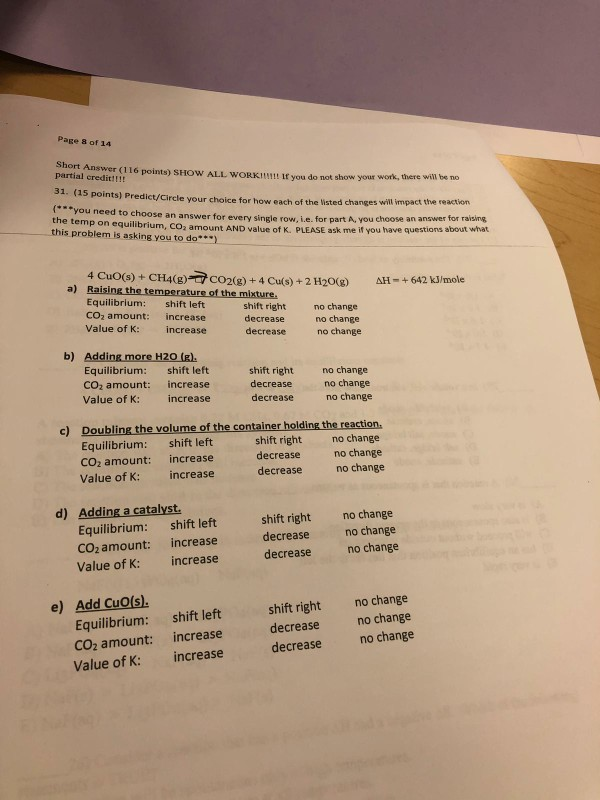pls do all Page 8 of 14 Short Answer (116 points) SHOw ALL wORKI!!!!I if you do not show your partial eredit!!!! work, there will be no 31. (15 points) Predict/Circle your choice for how each of the l isted changes will mpact the reaction you need to choose an the temp on equilibrium, this problem is asking you to do) answer for every single row, i.e. for part A, you choose an answer for raising CO2 amount AND value...

• ### 6) Which of the following statements is true? A) If Q = K, it means the reaction is not at equilibrium B) If Q<...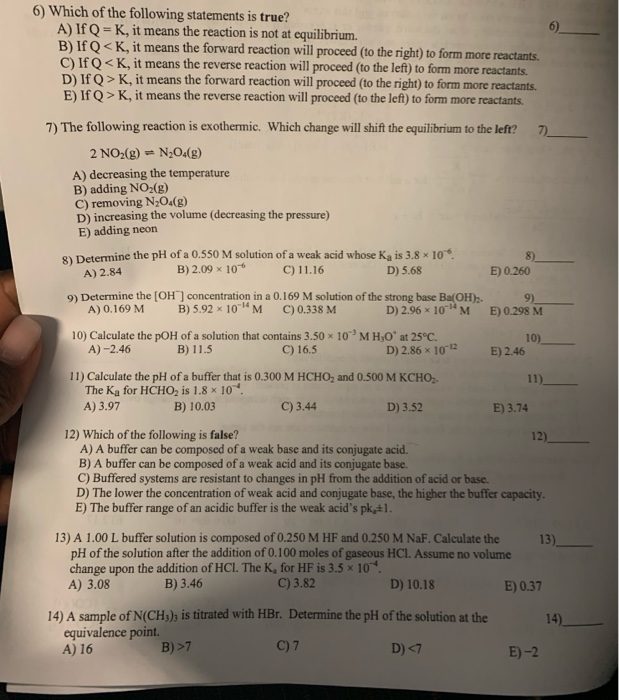6) Which of the following statements is true? A) If Q = K, it means the reaction is not at equilibrium B) If Q< K, it means the forward reaction will proceed (to the right) to form more reactants. C) If Q< K, it means the reverse reaction will proceed (to the left) to form more reactants. D) If Q> K, it means the forward reaction will proceed (to the right) to form more reactants E) If Q> K, it...

• ### answer all 6) cernents is true? equilibrium co the right 5) Determine the value of Ke...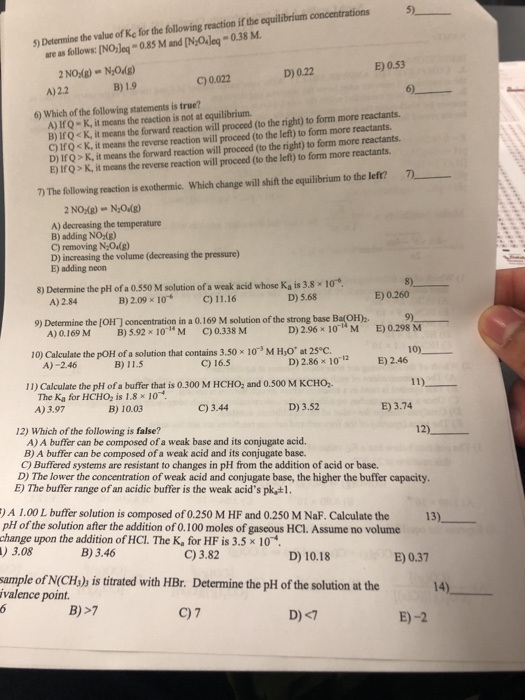answer all 6) cernents is true? equilibrium co the right 5) Determine the value of Ke for the following reaction if the equilibrium concentrations are as follows: (NO Joy -0.85 M and [NO_Jeg -0.38 M. 2 NO:(8) - N,Od D) 0.22 E) 0.53 A) 2.2 C) 0.022 B) 19 6) Which of the following statements is true? A) 10-K, it means the reaction is not at equilibrium. B) TOK, it means the forward reaction will proceed to the right to...

Need Online Homework Help?